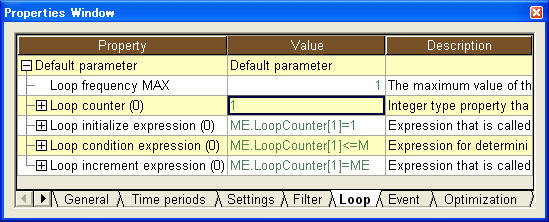Repeating a command

You can specify conditions in a command to automatically repeat (loop) the command.

Loop properties

Loop-related properties are available for commands. You can find those properties in the Loop tab of the properties window.Loop frequency MAX

The maximum value of the number of loops. Even when the loop condition expression is TRUE, the loop will stop if the number of loops exceeds this value. If no value is specified for this property, the value will be regarded as 1.

Loop counter

You can use this property as a counter for repeating a command. You can specify multiple values.

Loop initialize expression

This expression is executed only once when the loop starts. You can specify multiple expressions. When multiple expressions are specified, all the expressions will be executed. The default value is
ME.LoopCounter=1

Loop condition expression

Specify here loop conditions. You can specify multiple expressions. When multiple expressions are specified, all the expressions will be executed. If the result of the condition expression is TRUE, the command will be executed. The default value is
ME.Command_LoopCounter<=ME.Command_LoopMaxFrequency

You will use the property "Loop frequency MAX" if you want a loop to repeat for a certain number of times and the property "Loop condition expression" if you cannot define the number of loops. In the latter case, please specify a large number for the loop frequency MAX (i.e., a number larger than the number of times the loop is expected to be repeated with the loop condition expression).

Loop increment expression

This expression is executed after executing the command. You can specify multiple expressions. When multiple expressions are specified, all the expressions will be executed. The default value is
ME.LoopCounter=ME.LoopCounter+1

The flow of the loop process for a command is as follows.

1. Executes the loop initialize expression.
2. Executes the loop condition expression to confirm loop conditions.
• Will terminate without executing the command if the number of loops exceeds the loop frequency MAX.
• Will execute the command if the results of evaluation of all the loop condition expressions are TRUE.
• Will terminate without executing the command if the results of evaluation of any of the loop condition expressions are FALSE.
3. Executes the loop increment expression.
4. Returns to the second process.

As you can see from the flow of the process, the default value is set to execute the command only once.

Note

- If any of the expressions cannot be executed, the process will terminate at that point and the next command will not be executed.

- Currently for commands, the loop works only for scheduling parameters.

- Each of the following items is determined before the loop begins. Even if you change any of them during the loop, it will not affect the loop at that time.

- Loop repetitions MAX

- Loop initialize expression

- Loop condition expression

- Loop increment expression

Example

We will divide a four-week schedule into one week each and assign due date of each order forward from the beginning of each week.

Setting a loop

Set the loop frequency MAX as follows so that the loop occurs four times.

 Loop frequency MAX 4

Assignment start time

Using the loop counter, specify an expression for the assignment start time so that the orders will be assigned from the beginning of each week.

 Assignment start time PROJECT.SchedulingStartTime+(1w*(PROJECT.CommandReschedule.LoopCounter-1))

Filter orders

Using the loop counter, specify an expression to filter orders of which the due dates are within the assignment period.

 Filter orders ME.LET>=PROJECT.BasisTime+(1w*(PROJECT.CommandReschedule.LoopCounter-1))&&ME.LET